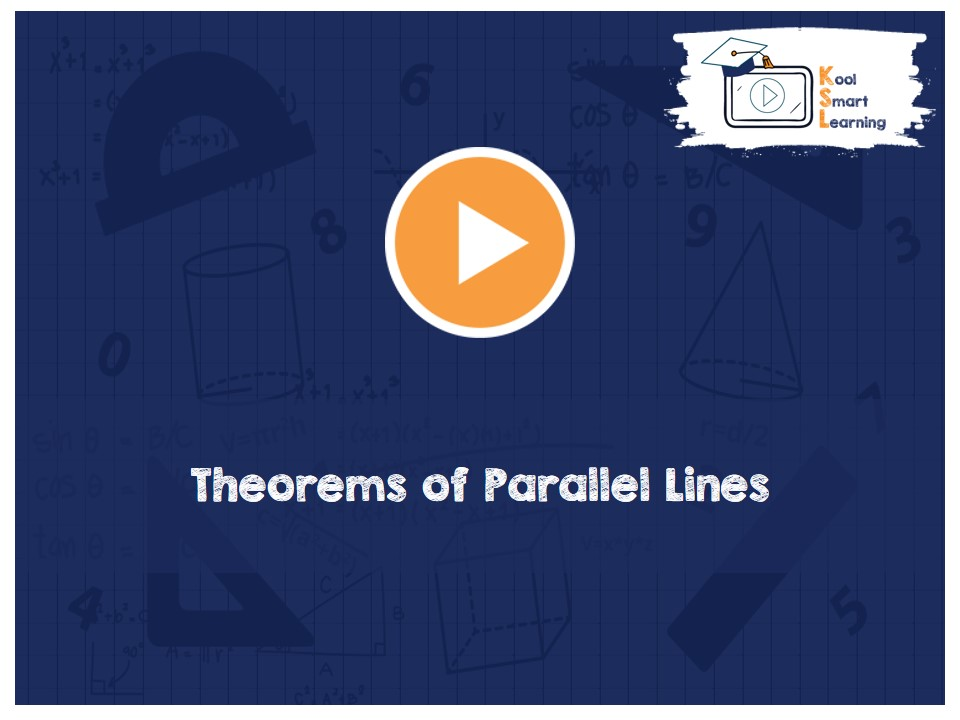At KoolSmartLearning, we intend to harness the power of online education to make learning easy.## Theorems of Parallel Lines

/  Theorems of Parallel Lines## Theorems of Parallel Lines

This video talks about the Theorems of the Parallel Lines and Transversal in the Lines and Angles topic. The theorems covered in this video are -(i) If a transversal intersects two parallel lines, then each of alternate interior angles is equal and its converse theorem (ii) If a transversal intersects two parallel lines, then each pair of interior angles on the same side of the transversal is supplementary and its converse theorem (iii) Lines which are parallel to the same line are parallel to each other. It also discusses the different conditions which can be checked to find out whether the given lines are parallel lines or not.

More Related Videos